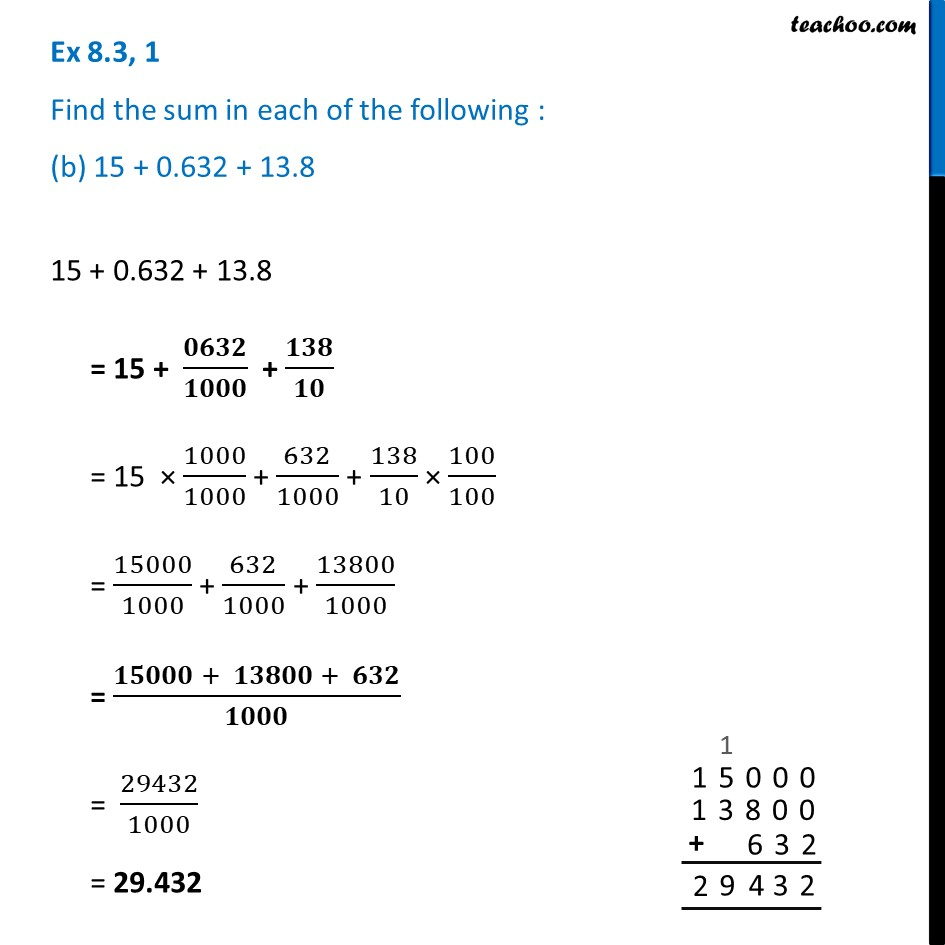Ex 8.3

Chapter 8 Class 6 Decimals
Serial order wiseLearn in your speed, with individual attention - Teachoo Maths 1-on-1 Class

### Transcript

Ex 8.3, 1 Find the sum in each of the following : (b) 15 + 0.632 + 13.815 + 0.632 + 13.8 = 15 + 𝟎𝟔𝟑𝟐/𝟏𝟎𝟎𝟎 + 𝟏𝟑𝟖/𝟏𝟎 = 15 × 1000/1000 + 632/1000 + 138/10 × 100/100 = 15000/1000 + 632/1000 + 13800/1000 = (𝟏𝟓𝟎𝟎𝟎 + 𝟏𝟑𝟖𝟎𝟎 + 𝟔𝟑𝟐)/𝟏𝟎𝟎𝟎 = 29432/1000 = 29.432